# Introduction To Three Dimensional Geometry NCERT Solutions : Class 11 Maths

## NCERT Solutions for Class 11 Maths : Introduction To Three Dimensional GeometryFilter
Filters :
Classes
•
•
•
•
•
•
•
Chapters
•
•
•
•
•
•
•
•
•
•
• 6 More
Exercises
•
•
•
•
•

Doubtnut one of the best online education platform provides free NCERT Solutions of Maths for Class 11 Introduction To Three Dimensional Geometry which are solved by our maths experts as per the NCERT (CBSE) guidelines. We provide the solutions as video solutions in which concepts are also explained along with answer which will help students while learning , preparing for exams and in doing homework. These Solutions will help to revise complete syllabus and score more marks in examinations.

Get here free, accurate and comprehensive NCERT Solutions for Class 11 Maths Introduction To Three Dimensional Geometry which have been reviewed by our maths counsellors as per the latest edition following up the CBSE guidelines. We provide video solutions in which solutions to all the questions of NCERT Class 11 Maths textbook are explained in step by step and detailed way.

## The Topics of the Chapter Introduction To Three Dimensional Geometry are :

QUESTIONS

GENERAL EQUATION OF CIRCLE

DISTANCE BETWEEN TWO POINTS

AREA OF A TRIANGLE

SECTION FORMULA

DISTANCE OF A POINT FROM A LINE

COORDINATE AXES AND COORDINATE PLANES IN THREE- DIMENSIONAL SPACE

It contains these exercises along with solved examples. All the exercises are solved in the video. Select the exercise to view the solutions exercisewise of the Chapter Introduction To Three Dimensional Geometry:

## NCERT Solutions Class 11 Maths Chapter Introduction To Three Dimensional Geometry Exercises:

We have covered all the exercises and also Solved examples in the videos. Along with the practise exercise Students should also practise solved examples to clear the concepts of the Introduction To Three Dimensional Geometry. If incase you have any doubt you can watch the solutions for the given questions.Watch the solutions for the given questions in which it is also explained in the video steps to solve the questions along with answers.

In this chapter, we will get to know about coordinate axes and coordinate planes, coordinates of a point in space, distance between two points and so on. Having a clear idea on how to tackle different questions is quite important. Doubtnut has introduced a lot of examples so that the students are able to get the perfect idea on how to solve the complex problems. By clearing all their doubts in a comprehensive and swift manner, students are able to achieve success in the examinations.

NCERT textbooks are found to be useful for the students to clear all the basic concepts. CBSE board adheres to NCERT textbook for its curriculum. However, students fail to attempt some of the tricky or complex questions in the exams. The reason behind this is the fact that they are unable to get an in-depth understanding of the subject. Math is a subject that require rigorous practice to get the proper idea on how to come up with the accurate solution in a timely manner. They should also be able to have a look at the different examples that would help to find the ultimate information about it. This, in turn, makes it possible to score good marks in the examination.  There are also lots of theorems in Geometry and unless they get the proper concept on how to work on it, things would become quite complicated as well.

Owing to the need for a good helping hand for the students of Maths, a team of the best and professional tutors of Doubtnut has introduced the most helpful video tutorials. Students can access online study materials without paying any money at all. In other words, the video tutorials can be accessed anytime and anywhere at zero cost. Therefore, students regardless of their financial background can access the quality study material to learn and excel in their respective exams. Moreover, Class 11 Maths NCERT Solutions chapter 12 Three Dimensional Geometry are helpful for students preparing for competitive exams like JEE Mains, JEE Advanced, BITSAT etc. It aids in quick revision as well. It is also possible to get the perfect support from the team of Doubtnut in case of any queries that arise in the minds of the students. So, it can really help in the progress of the students in the exams without any worry.

## Topics included NCERT Class 11 Maths Solutions Chapter 12 Introduction to Three Dimensional Geometry

### Introduction

You have learned that two intersecting mutually perpendicular lines in the plane are needed in order to locate the position of a plane. These are known as coordinate axes. In this chapter, we will learn the basic concepts of geometry in three-dimensional space.

### Coordinate Axes and Coordinate Planes in Three Dimensional Space

Let us consider three planes that intersect at a point O where the three planes happen to be mutually perpendicular to each other (look at the figure below.)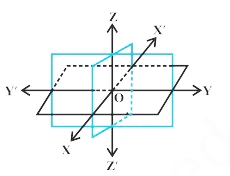You can find that these three planes intersect along the lines X′OX, Y′OY and Z′OZ, called the x, y, and z-axes, respectively. We may note that these lines are mutually perpendicular to each other. These lines constitute the rectangular coordinate system. The three coordinate planes are XY-plane, YZ-plane and the ZX-plane for the planes XOY, YOZ and ZOX respectively. Here we take the plane of the paper as the XOY plane and the line Z’OZ as perpendicular to the plane XOY. Here, it should be noted that the line Z’OZ will be vertical if we consider the plane of the paper as horizontal. When it comes to the origin of the coordinate system, it is the point O. The space is divided into eight parts by the three coordinate planes which is known as octants. The different names that these octants can be named are XOYZ, X′OYZ, X′OY′Z, XOY′Z, XOYZ′, X′OYZ′, X′OY′Z′ and XOY′Z′. It can also be denoted by I, II, III…respectively.

### Coordinates of a Point in Space

Here, we will have a look as to how it is possible to look forward to the right coordinates of a point in a space. This can be done by associating with three coordinates (x, y, z).  Look at the figure below: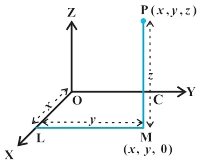In the point P in space, let us drop a perpendicular PM on the XY-plane with M as the foot of this perpendicular. Now we draw a perpendicular ML from the point M to the x-axis where it meets at L. Let OL be x, LM be y and MP be z. Then x, y and z coordinates are respective ones of x, y and z. In the figure, we may note that the point P (x, y, z) lies in the octant XOYZ and so all x, y, z are positive. Here, the signs of x, y and z would change in case P was in any other octant. So, to each point P in the space there corresponds an ordered triplet (x, y, z) of real numbers.

Conversely, given any triplet (x, y, z), we would first fix the point L on the x-axis corresponding to x, then locate the point M in the XY-plane. Here, it should be mentioned that the coordinates of the point M in the XY-plane are (x, y.) It can also be seen that LM is perpendicular to the x-axis. Here we draw a perpendicular line MP to the XY-plane and then try to make a location to the point P corresponding to z.  Thus (x, y, z) are the coordinates of the point P.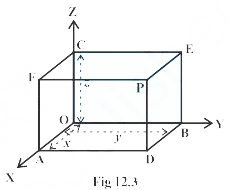Alternatively, through the point P in the space, we draw three planes parallel to the coordinate planes, meeting the x-axis, y-axis and z-axis in the points A, B and C, respectively (Fig 12.3). Let OA = x, OB = y and OC = z. Then, the point P will have the coordinates x, y and z and we write P (x, y, z). Here, we can locate the three points A, B and C with the given x, y and z. Through the points A, B and C we draw planes parallel to the YZ-plane, ZX-plane and XY-plane, respectively.

Here P is the point of intersection of the three planes ADPF, BDPE and CEPF. It correspondents to the ordered triplet (x, y, z) Thus we find that if P (x, y, z) happens to be any point in the space, then the perpendicular distances are x, y and z from YZ, ZX and XY planes respectively.

Let’s look at an example:

Example: If P is (2, 4, 5), find the coordinates of F in the fig 12.3

Solution:  When we take the point F, the distance which is measured along OY = 0. So, here the coordinates of F are (2, 0, 5)

### Distance between Two Points

We have learned about the distance between two points in two-dimensional coordinate system. Let us now look at the three-dimensional system.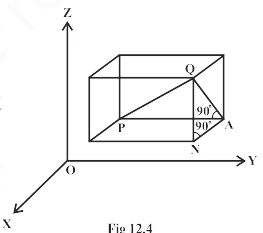Let P(x1, y1, z1) and Q (x2, y2, z2) be two points which is referred to a system of rectangular axes OX, OY and OZ. Now through the points P and Q, let’s draw planes parallel to the coordinate planes so as to form a rectangular parallelepiped with one diagonal PQ (Fig 12.4).

Now, since PAQ is a right angle, in triangle PAQ,

PQ2 = PA2 + AQ2  ………………  (1)

It is to be noted that triangle ANQ is right angle triangle with ANQ a right angle

Therefore AQ2 = AN2 + NQ2 ................ (2)

Now from (1) and (2), we have

PQ2 = PA2 + AN2 + NQ2

Now PA = y2 – y1, AN = x2 – x1 and NQ = z2 – z1

Hence PQ2 = (x2 – x1)2 + (y2 – y1) 2 + (z2 – z1)2

Therefore,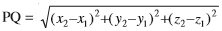So, here we get the distance between two points (x1, y1, z1) and (x2, y2, z2)

In particular, if x1 = y1 = z1 = 0, i.e., point P is origin O, then OQ =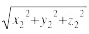This gives the distance between the origin O and any point Q (x2, y2, z2)

Let’s look at an example:

Example: Can you find out whether the points A (3, 6, 9), B (10, 20, 30) and C (25, – 41, 5), are the vertices of a right-angled triangle?

Solution By the distance formula, we have

AB2 = (10 – 3)2 + (20 – 6) 2 + (30 – 9) 2

= 49 + 196 + 441 = 686

BC2 = (25 – 10) 2 + (– 41 – 20) 2 + (5 – 30) 2

= 225 + 3721 + 625 = 4571

CA 2 = (3 – 25) 2 + (6 + 41) 2 + (9 – 5) 2

= 484 + 2209 + 16 = 2709

Her we can see that CA 2 + AB 2 ≠ BC 2.

Therefore, the triangle ABC is not a right-angled triangle.

### Section Formula

Here, we will learn in section formula how to extend the coordinates of the point to three-dimensional geometry.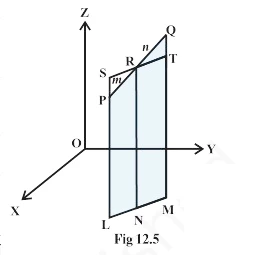There are two given points P (x1, y1, z1) and Q (x2, y2, z2). Let the point R (x, y, z) divide PQ in the given ratio m n internally. Now, let us draw PL, QM and RN perpendicular to the XY-plane. Obviously, PL || RN || QM and feet of these perpendiculars lie in a XY-plane. The points L, M and N will lie on a line which is the intersection of the plane containing PL, RN and QM with the XY-plane. Through the point R draw a line ST parallel to the line LM. Line ST will intersect the line LP externally at the point S and the line MQ at T, as shown in Fig 12.5.

It should be noted that quadrilaterals LNRS and NMTR are parallelograms. The triangles PSR and QTR are similar. Therefore,

m = PR = SP = SL – PL = NR – PL = z – z1

n    QR    QT    QM – TM   QM – NR   z2 - z

Therefore, it implies z = mz2 + nz1

m + n

Here, if we draw perpendiculars to the XZ and YZ-planes, we get

y = my2 + ny1 and x = mx2 + nx1

m + n                    m + n

Hence, the coordinates of the point R which divides the line segment joining two points P (x1, y1, z1) and Q (x2, y2, z2) internally in the ratio m:n are

mx2 + nx1, my2 + ny1, mz2 + nz1

m + n         m + n       m + n

If PQ is divided by the point R externally in the ratio m:n, then its coordinates are obtained by replacing n by – n so that coordinates of point R will be

mx2 - nx1, my2 - ny1, mz2 - nz1

m - n         m - n       m – n

Example:  Look out for the coordinates of the point which divides the line segment joining the points (1, –2, 3) and (3, 4, –5) in the ratio 2 : 3 (i) internally, and (ii) externally.

Solution:  (i) Let’s take P (x, y, z) be the point which divides the line segment joining A(1, – 2, 3) and  B (3, 4, –5) internally in the ratio 2 : 3. Therefore

x = 2(3) + 3(1) = 9

2 + 3          5

y = 2(4) + 3(–2) = 2

2 + 3          5

z = 2(–5) + 3(3) = –1

2 + 3          5

Thus, the required point is 9, 2, -1

5  5   5

(ii) Here, let’s assume P (x, y, z) to be the point which divides segment joining A (1, –2, 3) and B (3, 4, –5) externally in the ratio 2 : 3. Then

x = 2(3) + (–3)(1) = = -3

2 + (–3)

y = 2(4) + (–3)(–2) = = –14

2 + (–3)

z = 2(–5) + (–3)(3) = =19

2 + (–3)

Therefore, the required point is (–3, –14, 19).

## Role of Doubtnut for Class 11 Maths students

Doubtnut aims to provide the quality education to the students of any financial background. It is because the solutions at Doubtnut are provided at free of cost. So, let us have a look at some other advantages of learning via Doubtnut:

• Lots of examples and exercises included: There are lot of useful exercises and examples that help the students to clear their queries. Different type of questions helps the students in gaining the in depth understanding of a particular mathematical concept in a comprehensive manner
• Proves to be quite flexible: The study materials prove to be quite flexible as the students can access it from anywhere without any problem at all. The downloadable NCERT Solutions PDFs can be accessed anytime and anywhere.

Simple and lucid language: The video tutorials are curated and reviewed by experienced subject matter experts for presenting the solutions in simple, coherent and easy to understand language.

Share this on :-